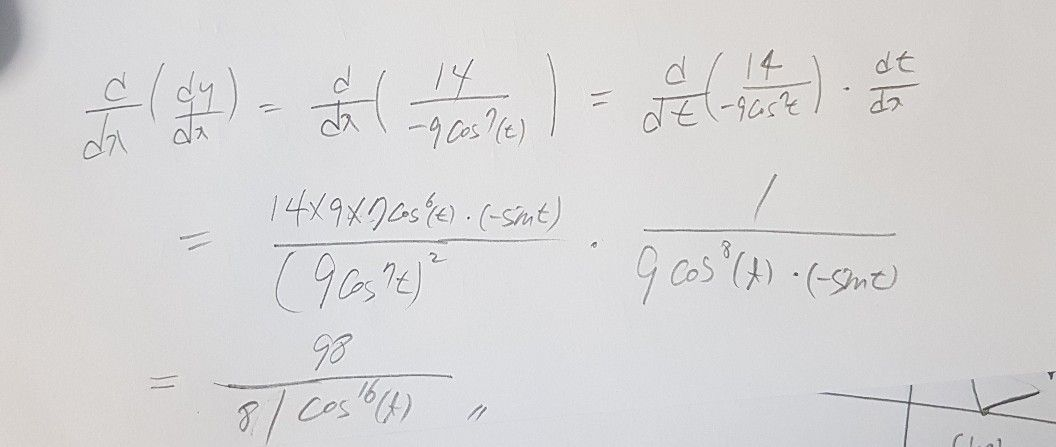수식부호
문제At least one of the answers above is NOT $CO\pi eCt$ $\left(1POin1\right)$ (a) 아래 주어진 매개변수방정식에서 $\dfrac {dy} {dx}$ 을 구하시오. $x=$ $cos^{9}\left(t\right)$ $7sin^{2}\left(t\right)$ $y=$ $\dfrac {dy} {dx}=\bar{141\left(-9cosn\left(7\right)\left(t\right)\right)}$ $\left(0\right)\dfrac {d^{2}y} {dx^{2}}$ 을 구하시오. $\dfrac {d^{2}y} {dx^{2}}=\bar{\left(14cos^{n2}\left(1\right)-1}$ $405211450\left(\left(2c05$ $\right)sn\left(\right)9cs3\left($
고등학교
미적분2
풀이콴다 선생님 - 수학푸는핑구아직도 궁금하다면?
콴다 선생님께 질문하기